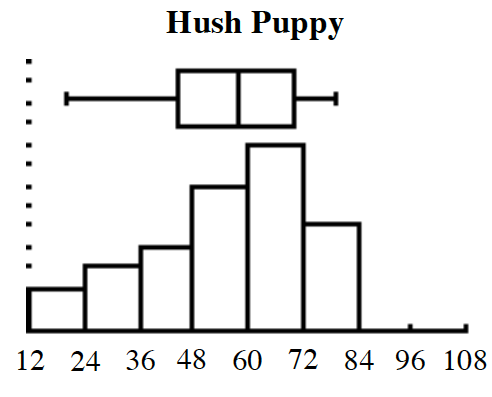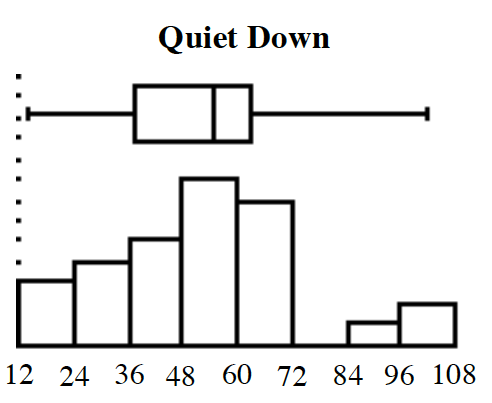### Home > INT3 > Chapter 2 > Lesson 2.1.1 > Problem2-12

2-12.

When vapor under high pressure is released into the air, the resulting noise can severely damage people’s hearing. This is a concern for large industrial facilities like power plants and factories. To help control this noise pollution, companies install silencers, which work on the same principles as car mufflers. Hector is an engineer for Vapor Kinetics. He is testing two different models of silencers, the Hush Puppy and the Quiet Down, by measuring the sound energy in decibels (dB) each silencer is producing at various temperatures and pressures. He has taken $30$ measurements for each silencer and graphed the two data sets.

1. Hector has calculated the five-number summaries for each data set, as shown below:

Hush Puppy: $\operatorname{min} = 19.7,$  $\text{Q}1 = 44.5,$ $\text{med} = 58.3,$ $\text{Q}3 = 70.1,$ $\text{max} = 79.5$
Quiet Down: $\text{min} = 14.2,$ $\text{Q}1 = 37.4,$ $\text{med} = 54.85,$ $\text{Q}3 = 63.3,$ $\text{max} = 102.1$

Using the graphs and the statistics, describe the center, shape, spread and outliers for each silencer.

Hush Puppy: The distribution is left skewed so its center and spread are best described by the median of $58.3$ dB and IQR of $25.6$ dB; there are no apparent outliers.

2. Hector needs to recommend one design for production. Which one do you suggest and why?

Which design has the lower median sound level?

3. The decibel scale is not linear. For example, a $105$ dB sound is $8$ times louder than a $75$ dB sound. This is roughly the difference in noise between running a kitchen blender and running a chain saw. Does this information change your answer to part (b)? Why or why not?